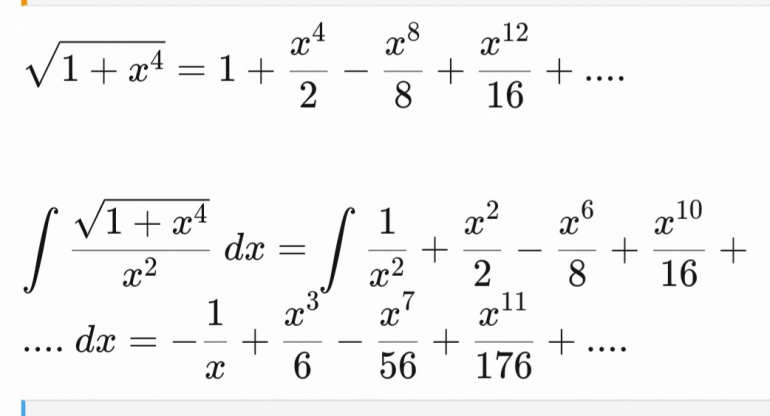unJonJoined: Jul 1, 2018
• Posts: 3552
July 29th, 2022 at 12:53:34 PM permalink
If you have wolfram alpha pro you can just type in arc length 1/X and click show step by step solution.
The race is not always to the swift, nor the battle to the strong; but that is the way to bet.
gordonm888Joined: Feb 18, 2015
• Posts: 3765
July 29th, 2022 at 2:38:04 PM permalink
Recognizing that this was either an unsolvable integral (i.e., no analytic form for the anti-derivative) or at least one for which the solution is not widely known, I was just typing in notes in my posts as I studied it. I realized that my posts were not directly helpful, sho88, I was just trying to come up with a good idea on how to start the analysis.

I did notice that the integral of (1/sqrt(a2+u2) = arsinh (u/a) + C This is the closest form that I have found for a simple integral relevant to your problem.

Also, the integral of(1/(u*sqrt(a2+u2)) = arcsch |(u/a)| + C where I think arcsch is an arc-hyperbolic cosecant.

All of which seems to be in sync with teliot's comment about Wolfram's advice to look at hyperbolic functions. However, hyperbolas have different mathematical properties than the curve you are studying and I'm not sure if they will reduce to your curve by, say, setting the distance between the two foci to 0.

Certainly not an "easy math problem."
So many better men, a few of them friends, are dead. And a thousand thousand slimy things live on, and so do I.
ssho88Joined: Oct 16, 2011
• Posts: 625
July 29th, 2022 at 6:19:44 PM permalink
Quote: gordonm888

Recognizing that this was either an unsolvable integral (i.e., no analytic form for the anti-derivative) or at least one for which the solution is not widely known, I was just typing in notes in my posts as I studied it. I realized that my posts were not directly helpful, sho88, I was just trying to come up with a good idea on how to start the analysis.

I did notice that the integral of (1/sqrt(a2+u2) = arsinh (u/a) + C This is the closest form that I have found for a simple integral relevant to your problem.

Also, the integral of(1/(u*sqrt(a2+u2)) = arcsch |(u/a)| + C where I think arcsch is an arc-hyperbolic cosecant.

All of which seems to be in sync with teliot's comment about Wolfram's advice to look at hyperbolic functions. However, hyperbolas have different mathematical properties than the curve you are studying and I'm not sure if they will reduce to your curve by, say, setting the distance between the two foci to 0.

Certainly not an "easy math problem."

Possible to solve this problem with Taylor series ?

Taylor series, (1+y)^0.5 = 1 +y/2 - y^2/8 + y^3/16 - (5*y^4)/128 + (14*y^5)/256 +. . . . . .

For my case, just replace y with 1/x^4 . . . .then the integration will be very easy !

Last edited by: ssho88 on Jul 29, 2022
gordonm888Joined: Feb 18, 2015
• Posts: 3765
July 30th, 2022 at 1:20:57 PM permalink
Nice. But with y = x^4, is there any convergence issue when x<1?
So many better men, a few of them friends, are dead. And a thousand thousand slimy things live on, and so do I.
teliotJoined: Oct 19, 2009
• Posts: 2375
July 30th, 2022 at 5:38:26 PM permalink
Quote: ssho88

Possible to solve this problem with Taylor series ?

Taylor series, (1+y)^0.5 = 1 +y/2 - y^2/8 + y^3/16 - (5*y^4)/128 + (14*y^5)/256 +. . . . . .

For my case, just replace y with 1/x^4 . . . .then the integration will be very easy !

For a numerical approximation, this is exactly what I would do.
End of the world website: www.climatecasino.net
ssho88Joined: Oct 16, 2011
• Posts: 625
July 30th, 2022 at 7:00:38 PM permalink
Quote: gordonm888

Nice. But with y = x^4, is there any convergence issue when x<1?

Convergence issue is my concern too. Thanks for your comment.
ssho88Joined: Oct 16, 2011
• Posts: 625
July 30th, 2022 at 7:01:58 PM permalink
Quote: teliot

Quote: ssho88

Possible to solve this problem with Taylor series ?

Taylor series, (1+y)^0.5 = 1 +y/2 - y^2/8 + y^3/16 - (5*y^4)/128 + (14*y^5)/256 +. . . . . .

For my case, just replace y with 1/x^4 . . . .then the integration will be very easy !

For a numerical approximation, this is exactly what I would do.

gordonm888Joined: Feb 18, 2015
• Posts: 3765
August 6th, 2022 at 5:14:38 AM permalink
Did the Taylor series converge? Were you able to solve this integral?
So many better men, a few of them friends, are dead. And a thousand thousand slimy things live on, and so do I.
ssho88Joined: Oct 16, 2011
• Posts: 625
August 6th, 2022 at 6:53:50 AM permalink
Here is the integral solution:-However, I think that series only converge if -1 < x < 1. So I think still not able to find length of arc 1/x from x = 1 to x = 3. Any comment?
unJonJoined: Jul 1, 2018
• Posts: 3552
August 6th, 2022 at 7:09:14 AM permalink
Quote: ssho88

Here is the integral solution:-However, I think that series only converge if -1 < x < 1. So I think still not able to find length of arc 1/x from x = 1 to x = 3. Any comment?